# 1 The notion of correlation (again)

In some sense, this whole course/quarter will be on the notion of correlation, which is why I want to spend some time on reminding us all on what that is/means.

Definition: Two variables `A` and `B` are correlated

• if knowing the value/range of `A` makes it easier to ‘predict’ (better) the value/range of `B` than if one doesn’t know the value/range of `A`;
• if knowing the value/range of `B` makes it easier to ‘predict’ (better) the value/range of `A` than if one doesn’t know the value/range of `B`.

Here is an example where knowing `A` (or `B`) does not help ‘predicting’ `B` (or `A`):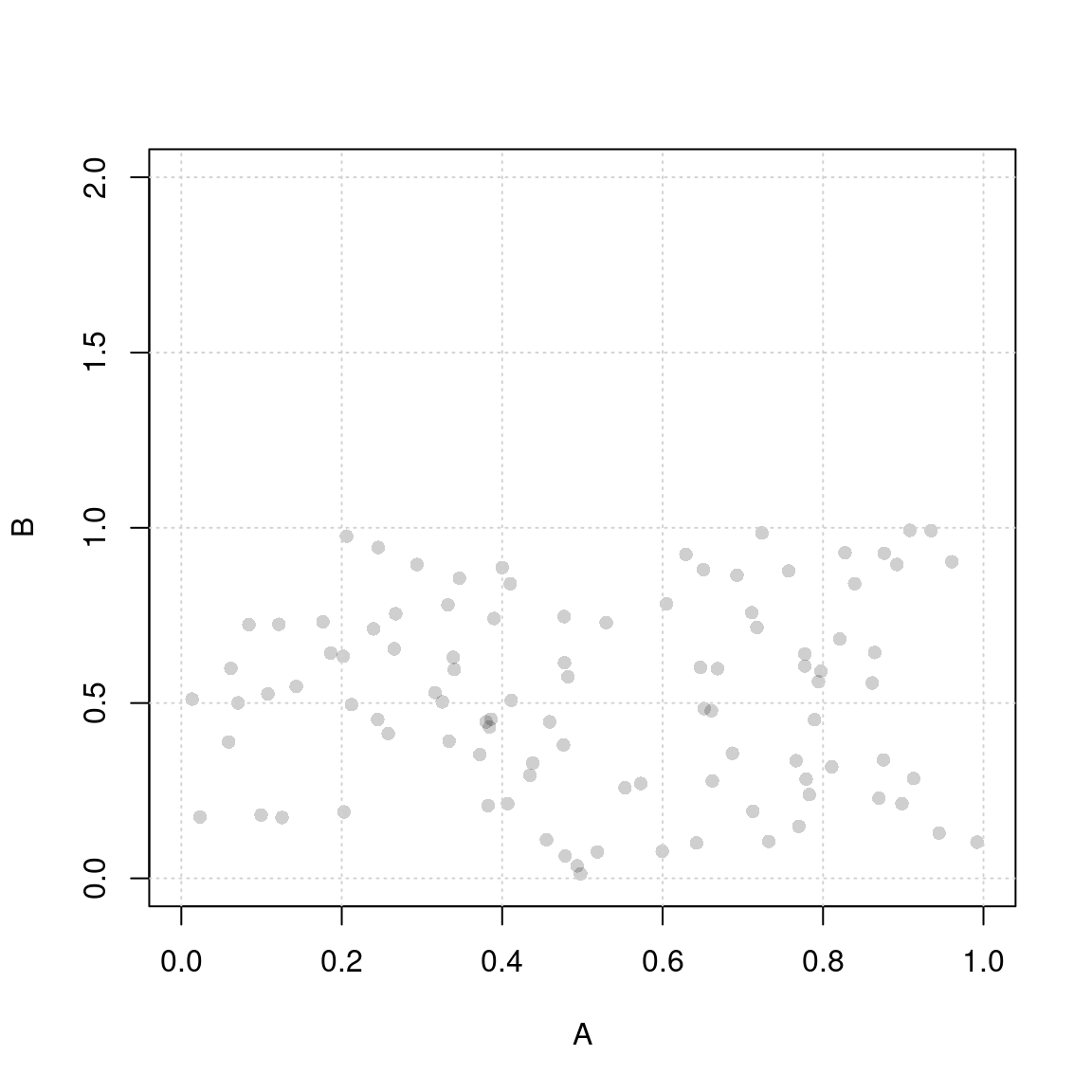``````##
##  Pearson's product-moment correlation
##
## data:  A and B
## t = 0.16863, df = 98, p-value = 0.8664
## alternative hypothesis: true correlation is not equal to 0
## 95 percent confidence interval:
##  -0.1799881  0.2127386
## sample estimates:
##        cor
## 0.01703215``````
``````##
## Call:
## lm(formula = B ~ A)
##
## Residuals:
##      Min       1Q   Median       3Q      Max
## -0.50405 -0.23895  0.00904  0.21547  0.46846
##
## Coefficients:
##             Estimate Std. Error t value Pr(>|t|)
## (Intercept)  0.50851    0.05974   8.512 2.03e-13 ***
## A            0.01730    0.10260   0.169    0.866
## ---
## Signif. codes:  0 '***' 0.001 '**' 0.01 '*' 0.05 '.' 0.1 ' ' 1
##
## Residual standard error: 0.2732 on 98 degrees of freedom
## Multiple R-squared:  0.0002901,  Adjusted R-squared:  -0.009911
## F-statistic: 0.02844 on 1 and 98 DF,  p-value: 0.8664``````

Why does knowing `A` (or `B`) not help ‘predicting’ `B` (or `A`)? Because, for instance, no matter which value range of `A` you pick, you can’t predict `B` very well (and vice versa):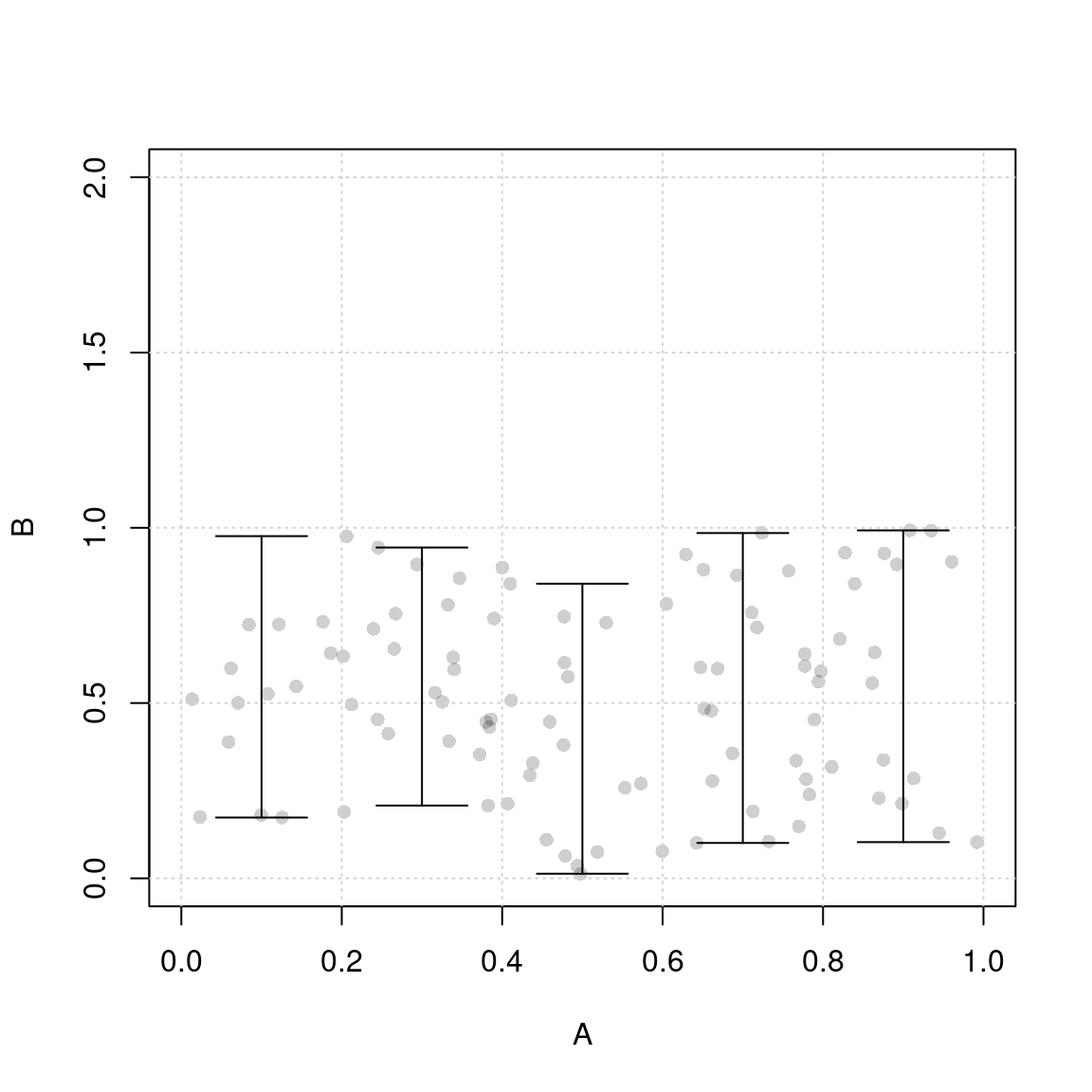And we can exemplify that easily with a regression line as well: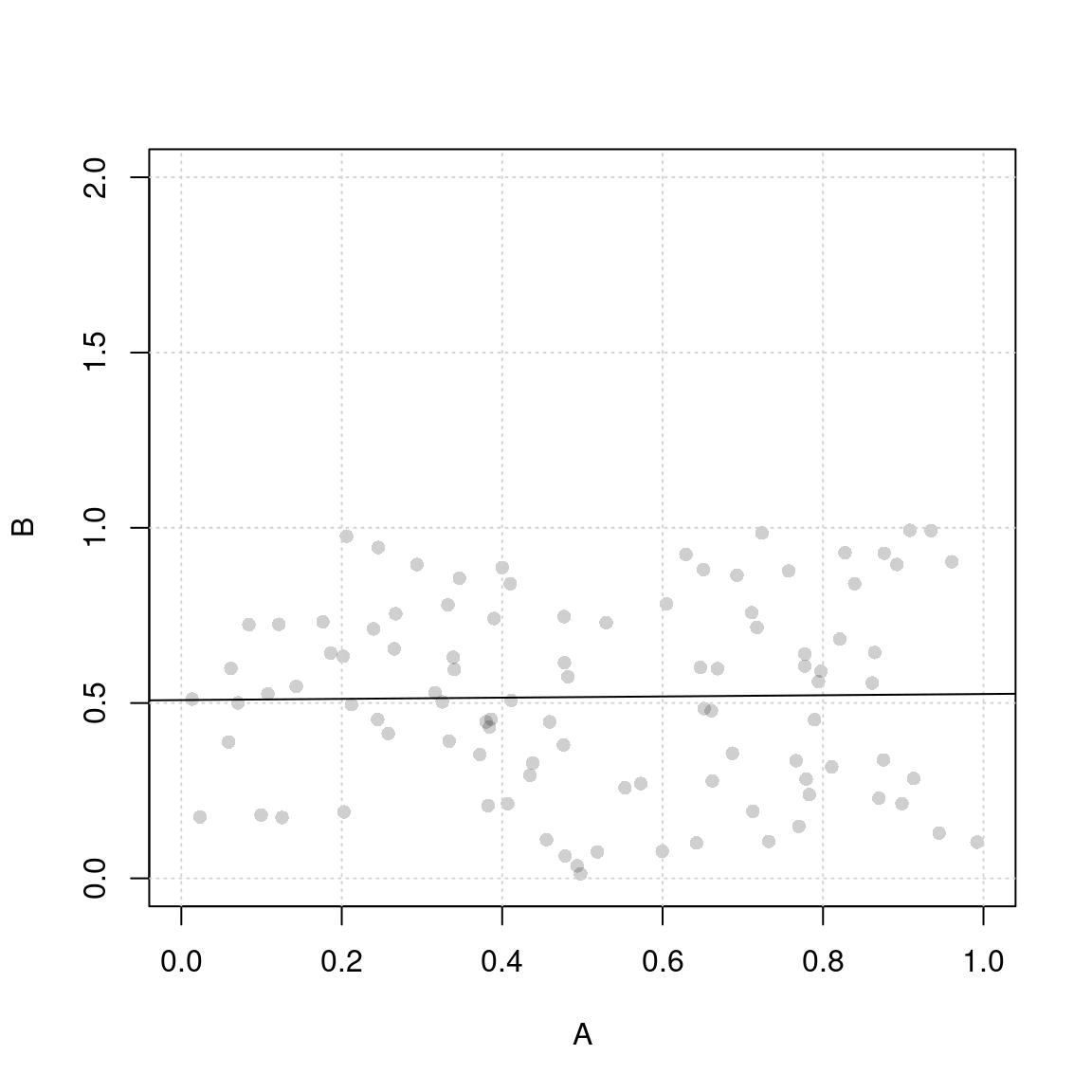By contrast, here is an example where knowing `A` (or `B`) does help ‘predicting’ `B` (or `A`):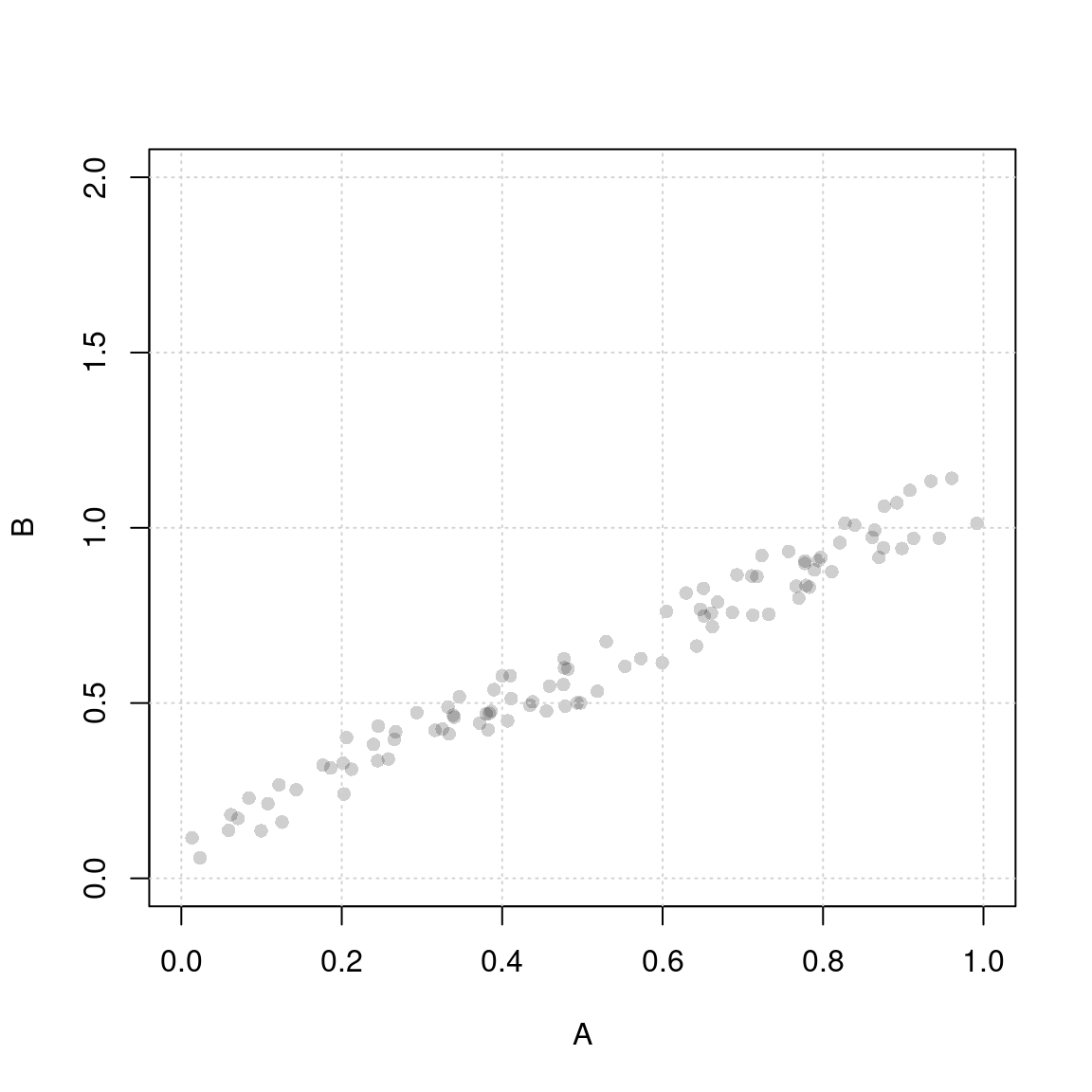``````##
##  Pearson's product-moment correlation
##
## data:  A and B
## t = 48.903, df = 98, p-value < 2.2e-16
## alternative hypothesis: true correlation is not equal to 0
## 95 percent confidence interval:
##  0.9705438 0.9866034
## sample estimates:
##       cor
## 0.9801194``````
``````##
## Call:
## lm(formula = B ~ A)
##
## Residuals:
##       Min        1Q    Median        3Q       Max
## -0.100809 -0.047789  0.001807  0.043093  0.093692
##
## Coefficients:
##             Estimate Std. Error t value Pr(>|t|)
## (Intercept)  0.10170    0.01195   8.512 2.03e-13 ***
## A            1.00346    0.02052  48.903  < 2e-16 ***
## ---
## Signif. codes:  0 '***' 0.001 '**' 0.01 '*' 0.05 '.' 0.1 ' ' 1
##
## Residual standard error: 0.05463 on 98 degrees of freedom
## Multiple R-squared:  0.9606, Adjusted R-squared:  0.9602
## F-statistic:  2391 on 1 and 98 DF,  p-value: < 2.2e-16``````

Why does knowing `A` (or `B`) help ‘predicting’ `B` (or `A`)? Because, for instance, knowing the value range of `A` makes you predict `B` better (and vice versa):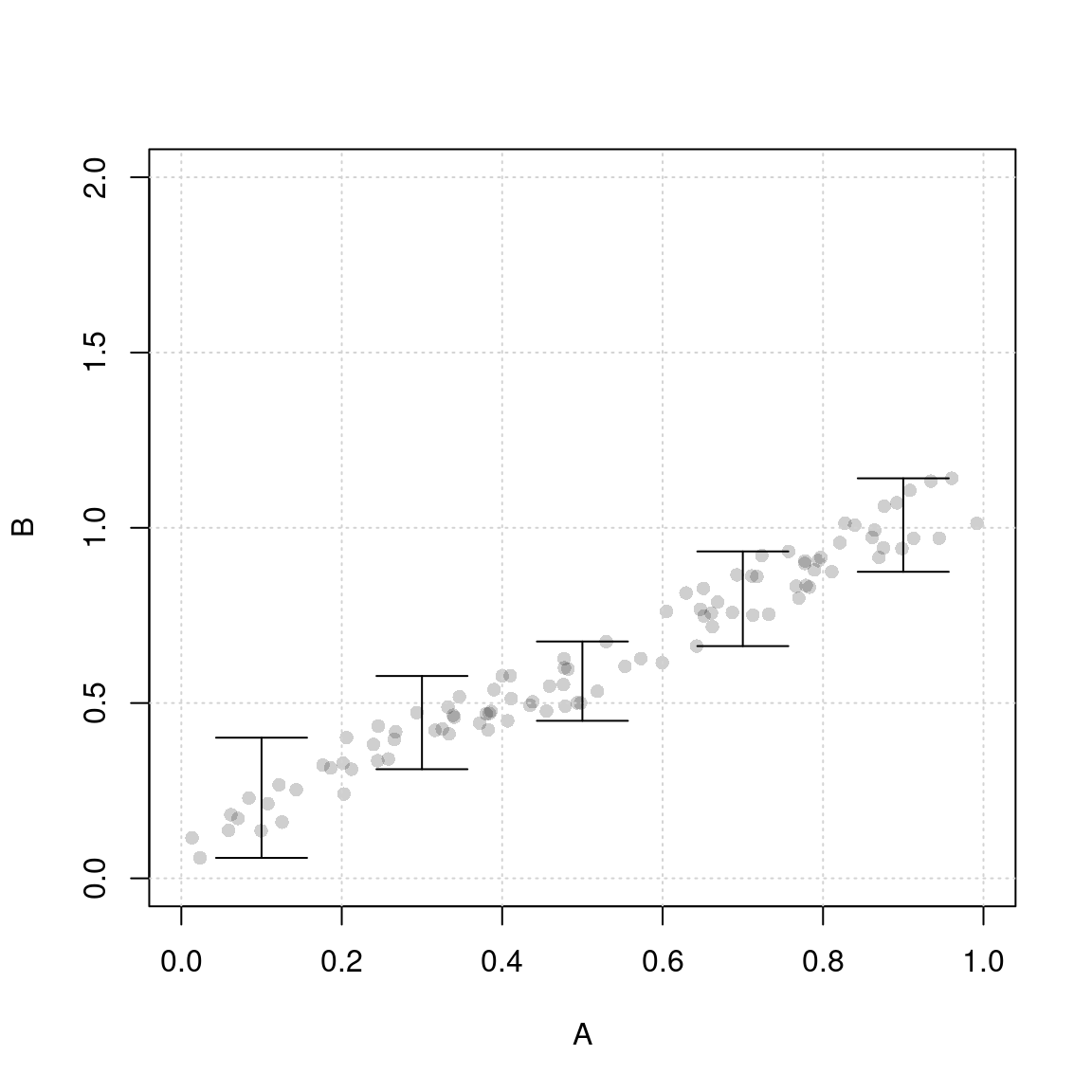And we can exemplify that easily with a regression line as well: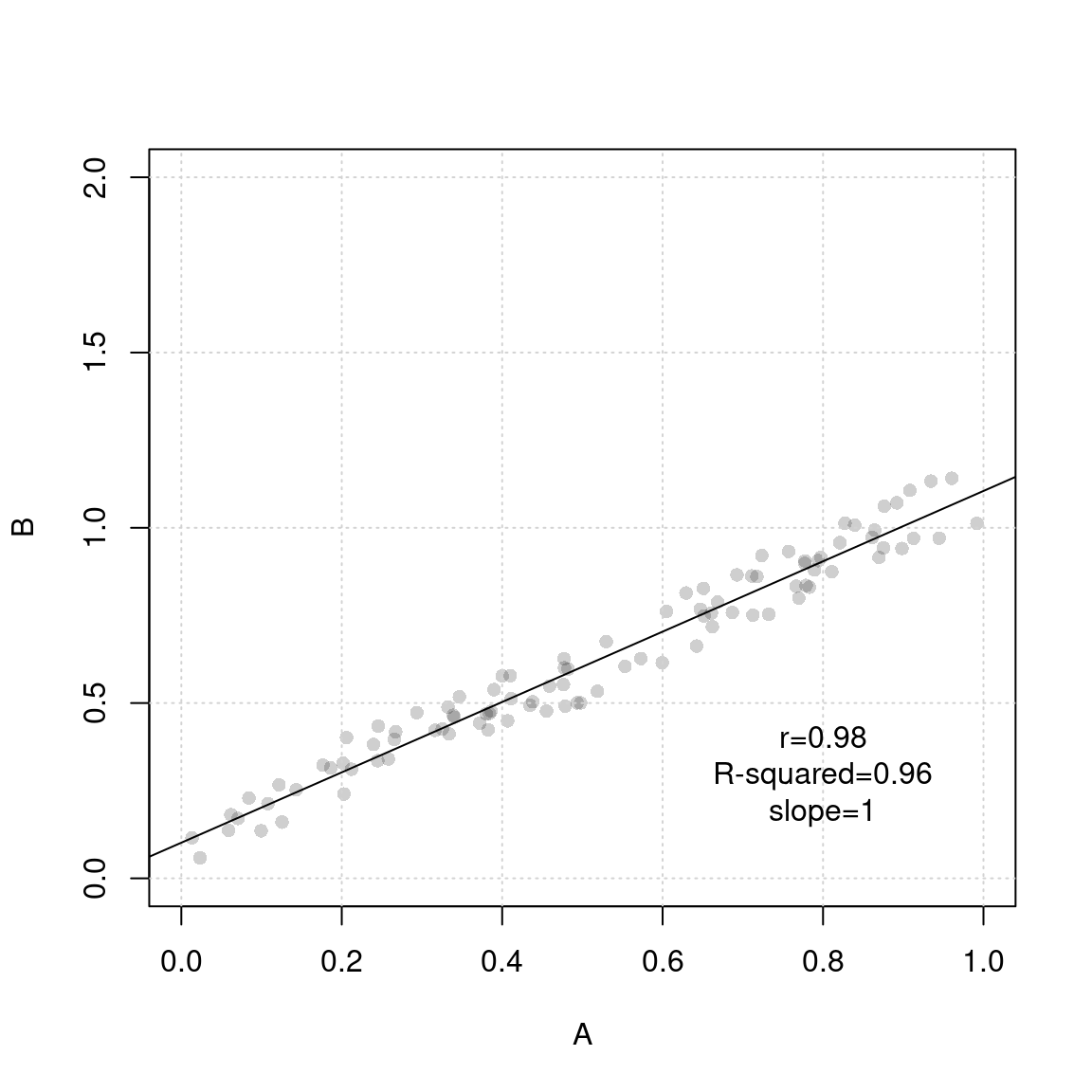Now, here’s an example of a correlation with the same slope, but more noise: